# 我花了一夜用数据结构给女朋友写个H5走迷宫游戏

17 篇文章 0 订阅

### 文章目录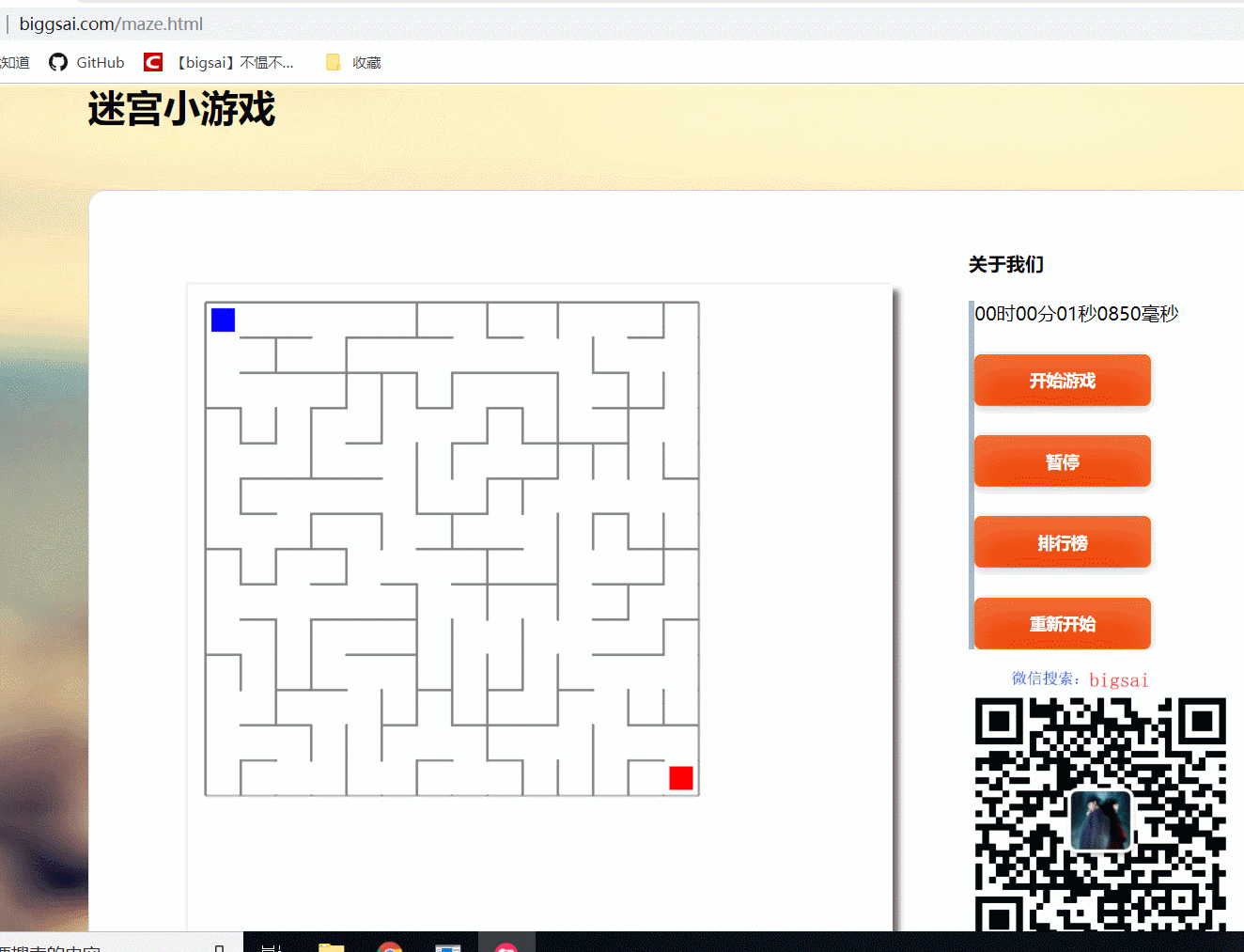# 起因# 分析

• 什么东西简单容易呢？我百度一下，我靠，这个鸟游戏原来不好搞啊，得接触一堆不熟悉的东西，搞不来搞不来。

• 超越妹妹又不是小孩子，糊弄不过去。

• 画线—>画迷宫(擦线)—>方块移动、移动约束(不出界不穿墙)—>完成游戏

# 画线(棋盘)

 <!DOCTYPE html>
<html>
<title>MyHtml.html</title>
<body>
<canvas id="mycanvas" width="600px" height="600px"></canvas>

</body>
<script type="text/javascript">

var aa=14;
var chess = document.getElementById("mycanvas");
var context = chess.getContext('2d');

//  var context2 = chess.getContext('2d');
//      context.strokeStyle = 'yellow';
var tree = [];//存放是否联通
var isling=[];//判断是否相连
for(var i=0;i<aa;i++){
tree[i]=[];
for(var j=0;j<aa;j++){
tree[i][j]=-1;//初始值为0
}
}  for(var i=0;i<aa*aa;i++){
isling[i]=[];
for(var j=0;j<aa*aa;j++){
isling[i][j]=-1;//初始值为0
}
}

function drawChessBoard(){//绘画
for(var i=0;i<aa+1;i++){
context.strokeStyle='gray';//可选区域
context.moveTo(15+i*30,15);//垂直方向画15根线，相距30px;
context.lineTo(15+i*30,15+30*aa);
context.stroke();
context.moveTo(15,15+i*30);//水平方向画15根线，相距30px;棋盘为14*14；
context.lineTo(15+30*aa,15+i*30);
context.stroke();
}
}
drawChessBoard();//绘制棋盘

//      var mymap=new Array(36);
//      for(var i=0;i<36;i++)
//     {mymap[i]=-1;}

</script>
</html>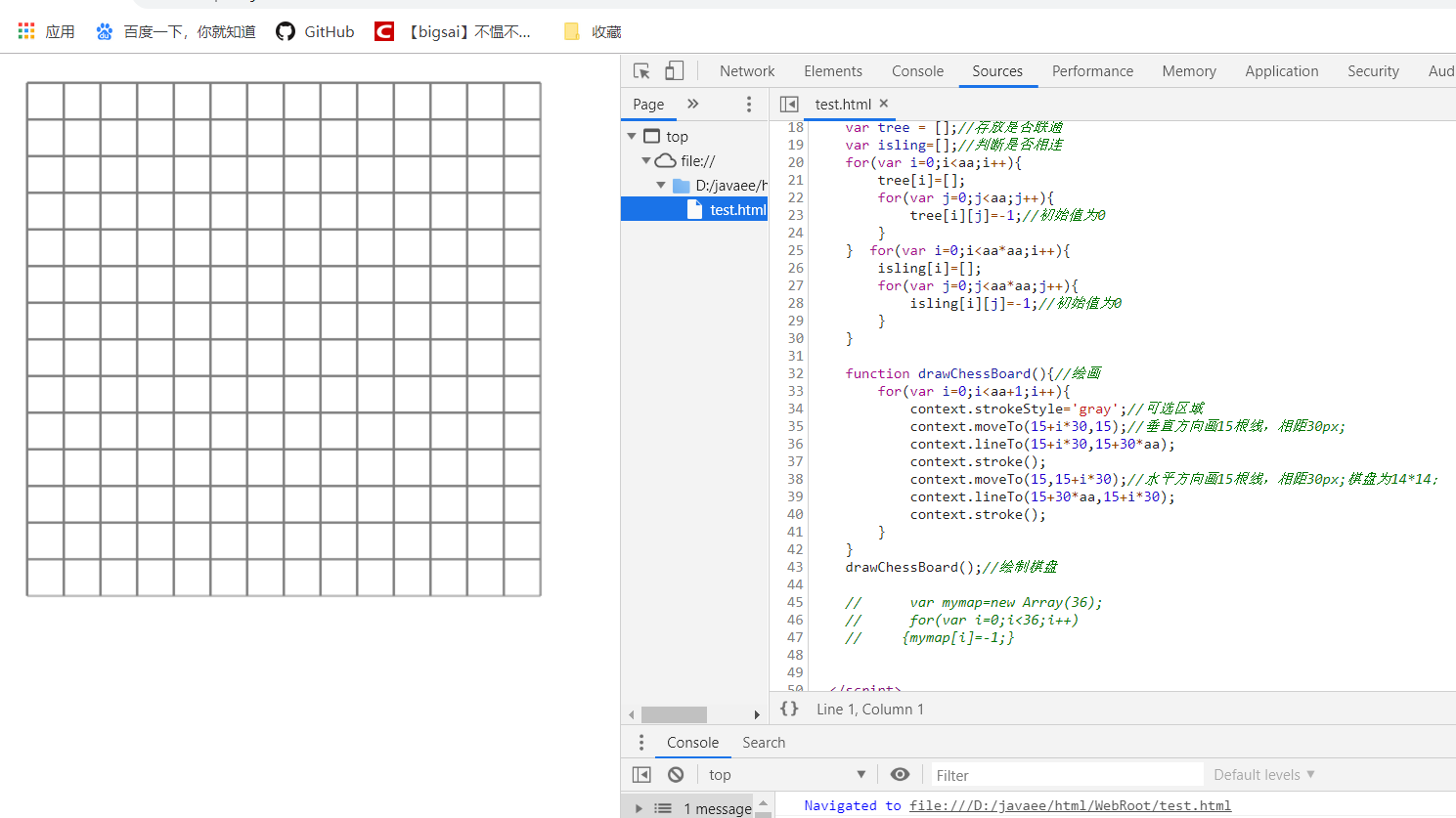# 画迷宫

• 因为我们想要迷宫，那么就需要这个迷宫出口和入口有连通路径，你可能压根不知道迷宫改怎么生成，用的什么算法。小声BB：并查集(不相交集合)

• 之前笔者在前面数据结构与算法系列中曾经介绍过并查集(不相交集合)，它的主要功能是森林的合并，不联通的通过并查集能够快速将两个森林合并，并且能够快速查询两个节点是否在同一个森林中！

我们的随机迷宫：在每个方格都不联通的情况下，是一个棋盘方格，这也是它的初始状态。而这个节点可以跟邻居可能相连，也可能不相连。我们可以通过并查集实现。

• 1：定义好不想交集合的基本类和方法（search,union等）
2：数组初始化，每一个数组元素都是一个集合，值为-1
3：随机查找一个格子（一维数据要转换成二维，有点麻烦），在随机找一面墙（也就是找这个格子的上下左右），还要判断找的格子出没出界。
具体在格子中找个随机数m——>随机数m在二维中的位置[m/长,m%长]——>这个二维的上下左右随机找一个位置p[m/长+1,m%长][m/长-1,m%长][m/长,m%长+1][m/长,m%长-1]——>判断是否越界
4：判断两个格子(一维数组编号)是否在一个集合(并查集查找)。如果在，则重新找，如果不在，那么把墙挖去
5：把墙挖去有点繁琐，需要考虑奇偶判断它那种墙(上下还是左右，还要考虑位置)，然后擦掉。（根据数组转换成真实距离）。具体为找一个节点，根据位置关系找到一维数组的号位用并查集判断是否在一个集合中。
6：最终得到一个完整的迷宫。直到第一个(1,1)和(n,n)联通停止。虽然采用随机数找墙，但是效果并不是特别差。其中要搞清一维二维数组的关系。一维是真实数据，并查集操作。二维是位置。要搞懂转化！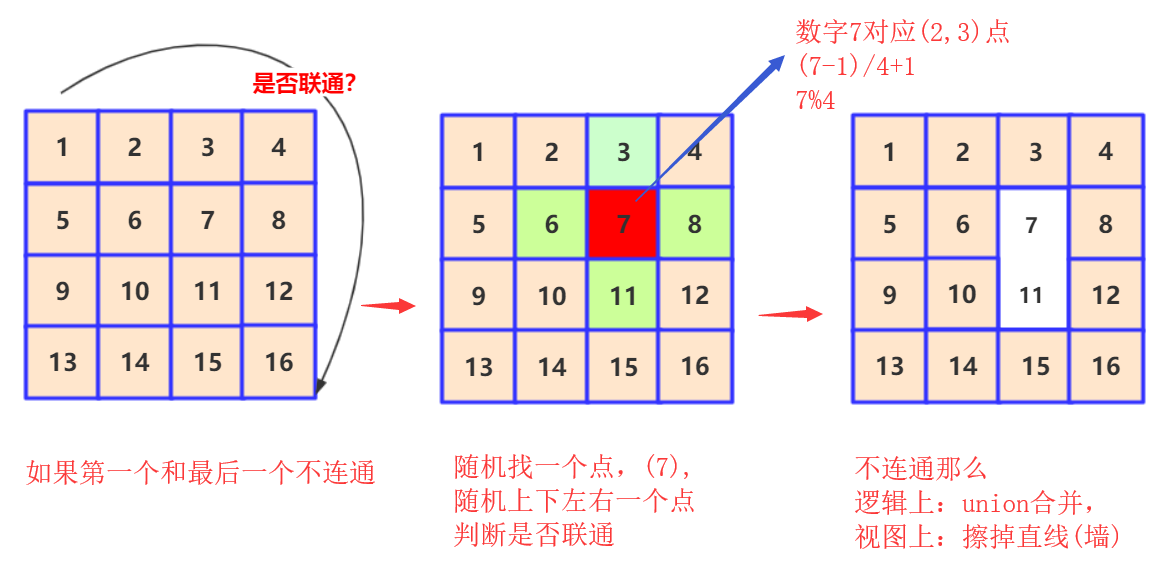while(search(0)!=search(aa*aa-1))//主要思路
{
var num = parseInt(Math.random() * aa*aa );//产生一个小于196的随机数
var neihbour=getnei(num);
if(search(num)==search(neihbour)){continue;}
else//不在一个上
{
isling[num][neihbour]=1;isling[neihbour][num]=1;
drawline(num,neihbour);//划线
union(num,neihbour);

}
}


<!DOCTYPE html>
<html>
<title>MyHtml.html</title>
<body>
<canvas id="mycanvas" width="600px" height="600px"></canvas>

</body>
<script type="text/javascript">
//自行添加上面代码
//      var mymap=new Array(36);
//      for(var i=0;i<36;i++)
//     {mymap[i]=-1;}
function getnei(a)//获得邻居号  random
{
var x=parseInt(a/aa);//要精确成整数
var y=a%aa;
var mynei=new Array();//储存邻居
if(x-1>=0){mynei.push((x-1)*aa+y);}//上节点
if(x+1<14){mynei.push((x+1)*aa+y);}//下节点
if(y+1<14){mynei.push(x*aa+y+1);}//有节点
if(y-1>=0){mynei.push(x*aa+y-1);}//下节点
var ran=parseInt(Math.random() * mynei.length );
return mynei[ran];

}
function search(a)//找到根节点
{
if(tree[parseInt(a/aa)][a%aa]>0)//说明是子节点
{
return search(tree[parseInt(a/aa)][a%aa]);//不能压缩路径路径压缩
}
else
return a;
}
function value(a)//找到树的大小
{
if(tree[parseInt(a/aa)][a%aa]>0)//说明是子节点
{
return tree[parseInt(a/aa)][a%aa]=value(tree[parseInt(a/aa)][a%aa]);//不能路径压缩
}
else
return -tree[parseInt(a/aa)][a%aa];
}
function union(a,b)//合并
{
var a1=search(a);//a根
var b1=search(b);//b根
if(a1==b1){}
else
{
if(tree[parseInt(a1/aa)][a1%aa]<tree[parseInt(b1/aa)][b1%aa])//这个是负数()，为了简单减少计算，不在调用value函数
{
tree[parseInt(a1/aa)][a1%aa]+=tree[parseInt(b1/aa)][b1%aa];//个数相加  注意是负数相加
tree[parseInt(b1/aa)][b1%aa]=a1;       //b树成为a树的子树，b的根b1直接指向a；
}
else
{
tree[parseInt(b1/aa)][b1%aa]+=tree[parseInt(a1/aa)][a1%aa];
tree[parseInt(a1/aa)][a1%aa]=b1;//a所在树成为b所在树的子树
}
}
}

function drawline(a,b)//划线，要判断是上下还是左右
{

var x1=parseInt(a/aa);
var y1=a%aa;
var x2=parseInt(b/aa);
var y2=b%aa;
var x3=(x1+x2)/2;
var y3=(y1+y2)/2;
if(x1-x2==1||x1-x2==-1)//左右方向的点  需要上下划线
{
//  context.beginPath();
context.strokeStyle = 'white';
//    context.moveTo(30+x3*30,y3*30+15);//
//   context.lineTo(30+x3*30,y3*30+45);
context.clearRect(29+x3*30, y3*30+16,2,28);
//    context.stroke();
}
else
{
//   context.beginPath();
context.strokeStyle = 'white';
//  context.moveTo(x3*30+15,30+y3*30);//
//    context.lineTo(45+x3*30,30+y3*30);
context.clearRect(x3*30+16, 29+y3*30,28,2);
//      context.stroke();
}
}

while(search(0)!=search(aa*aa-1))//主要思路
{
var num = parseInt(Math.random() * aa*aa );//产生一个小于196的随机数
var neihbour=getnei(num);
if(search(num)==search(neihbour)){continue;}
else//不在一个上
{
isling[num][neihbour]=1;isling[neihbour][num]=1;
drawline(num,neihbour);//划线
union(num,neihbour);

}
}
</script>
</html>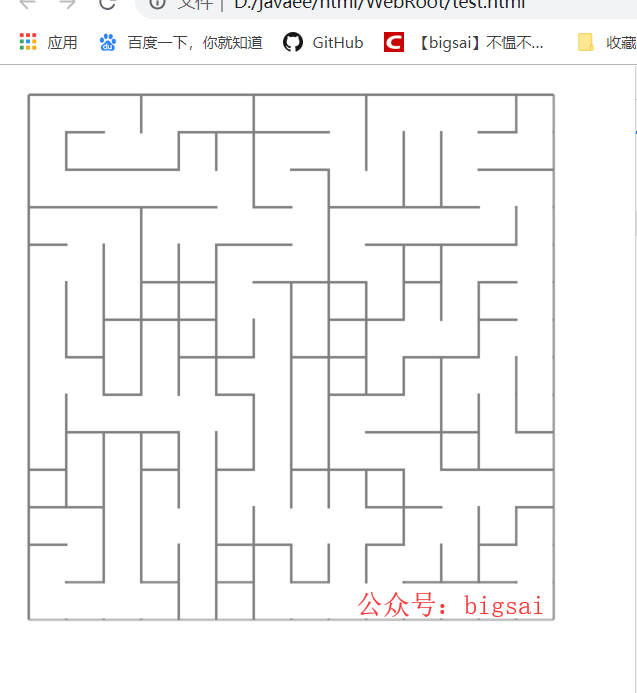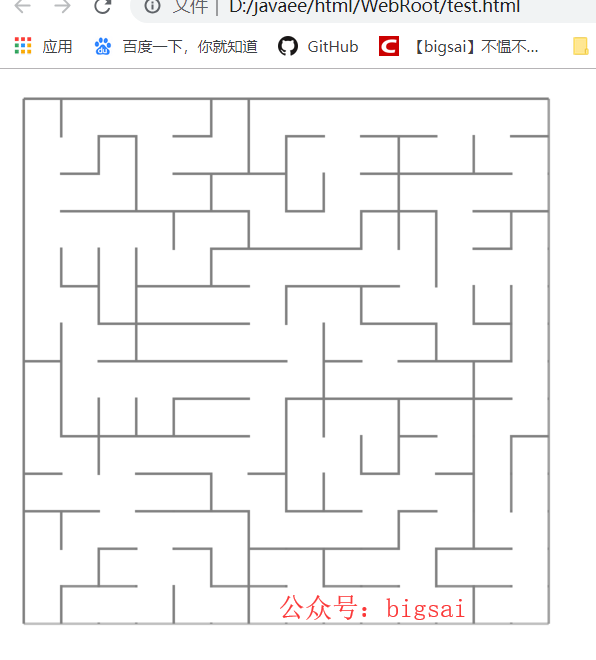# 方块移动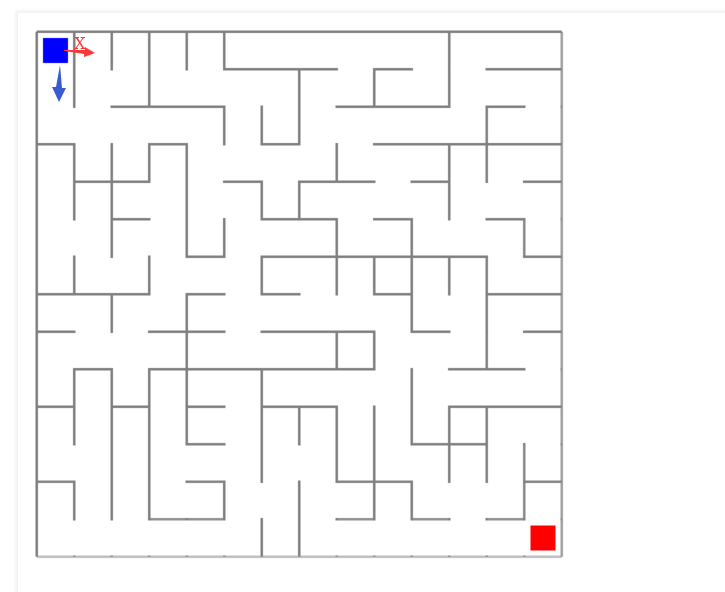# 结语05-1110-11
11-30101
04-191万+
06-011438
09-272238
12-22
04-25
01-01
05-18
10-28651
10-1321
07-26103点击重新获取扫码支付1.余额是钱包充值的虚拟货币，按照1:1的比例进行支付金额的抵扣。
2.余额无法直接购买下载，可以购买VIP、C币套餐、付费专栏及课程。余额充值